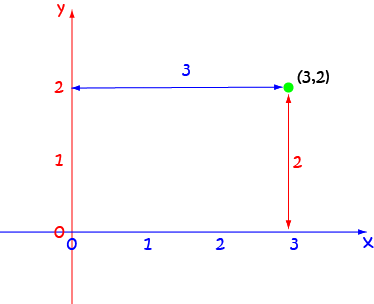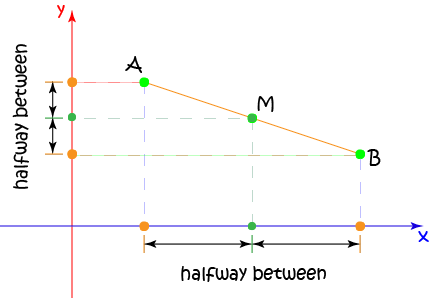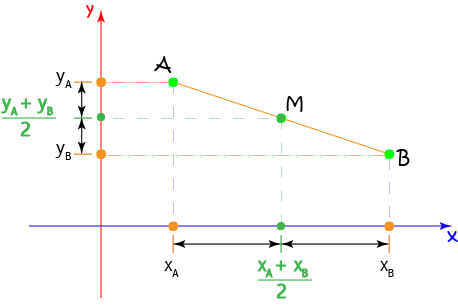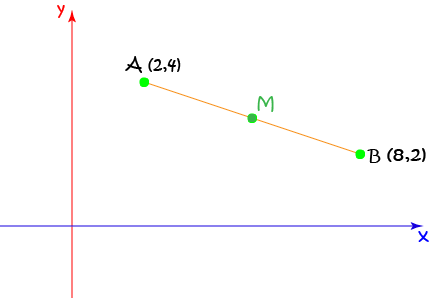# Midpoint of a Line Segment

The midpoint of a line segment is the point that is halfway between the two end points of the line segment. If you measure the line segment from each end to the midpoint, you end up measuring the same distance. The first step in finding the midpoint of a line segment, is to find the Cartesian coordinates for its end points. You will often be given this information, but let's start by reminding ourselves what Cartesian coordinates are.

## Cartesian Coordinates

The Cartesian coordinates of a point tell us where it lies in the $xy$-plane. They consist of an ordered pair $(x,y)$ that tells us how far across the plane and how far up the plane the point lies. The $x$-coordinate comes first, and tells us how far across the plane the point is. The $y$-coordinate comes second and tells us how far up the plane the point lies. In the picture, I've plotted the point $(3,2)$. It is three units across the plane and two units up the plane.## Finding the MidpointThe midpoint of the line segment $AB$ lies halfway between $A$ and $B$. So,

• Its $x$-coordinate is halfway between the $x$-coordinates of $A$ and $B$, and
• Its $y$-coordinate is halfway between the $y$-coordinates of $A$ and $B$.

To find the midpoint of $AB$:

1. Add the $x$-coordinate of $A$ to the $x$-coordinate of $B$ and divide by $2$ (take the average of the $x$-coordinates).
2. Add the $y$-coordinate of $A$ to the $y$-coordinate of $B$ and divide by $2$ (take the average of the $y$-coordinates).

The midpoint formula says:

$M = \left(\dfrac{x_A + x_B}{2}, \dfrac{y_A + y_B}{2} \right)$Let's finish with a couple of examples.

## Example 1Find the midpoint of the line segment $AB$ with endpoints $A(2,4)$ and $B(8,2)$.

Using the formula

\begin{align*} M &= \left(\dfrac{x_A + x_B}{2}, \dfrac{y_A + y_B}{2} \right)\\ &= \left(\dfrac{2 + 8}{2}, \dfrac{4 + 2}{2} \right)\\ &= \left(\dfrac{10}{2}, \dfrac{6}{2} \right)\\ &= (5,3) \end{align*}

## Example 2Find the midpoint of the line segment $AB$ with endpoints $A(-2,-1)$ and $B(8,4)$.

Using the formula

\begin{align*} M &= \left(\dfrac{x_A + x_B}{2}, \dfrac{y_A + y_B}{2} \right)\\ &= \left(\dfrac{-2 + 8}{2}, \dfrac{-1 + 4}{2} \right)\\ &= \left(\dfrac{6}{2}, \dfrac{3}{2} \right)\\ &= (3,1.5) \end{align*}

### Description

coordinate geometry is a branch of geometry where the position of the points on the plane is defined with the help of an ordered pair of numbers also known as coordinates. In this tutorial series, you will learn about vast range of topics such as Cartesian Coordinates, Midpoint of a Line Segment etc

### Audience

year 10 or higher, several chapters suitable for Year 8+ students.

### Learning Objectives

Explore topics related to Coordinates Geometry

Author: Subject Coach
You must be logged in as Student to ask a Question.